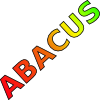#### You may also like### Consecutive Numbers

An investigation involving adding and subtracting sets of consecutive numbers. Lots to find out, lots to explore.### Tea Cups

Place the 16 different combinations of cup/saucer in this 4 by 4 arrangement so that no row or column contains more than one cup or saucer of the same colour.### Counting on Letters

The letters of the word ABACUS have been arranged in the shape of a triangle. How many different ways can you find to read the word ABACUS from this triangular pattern?

# 9 Weights

##### Age 11 to 14Challenge Level

Patrick from Woodbridge School gave a very clear explanation of how to work out which weight is heavier, in just two weighings of the balance, when you have been given 9 weights and you are told that one is slightly heavier than the rest:

It is always possible to find the different weight, using a simple method.
First, we think, "If we only had 2 weights, would this be possible in one weighing?". The answer is yes.
Then, "If we had three weights and one weighing, is this possible?" The answer is still yes, since we can put one weight on both sides of the balance. If they are equal then the unused weight is heavier, and if they are unbalanced then the side that goes down holds the heavier weight. Let us call this method Method 1.
We can divide the nine weights into three now:
1, 2, 3
4, 5, 6
7, 8, 9
BALANCE 456 and 789
If the weighings are equal then we can use Method 1 on weights 1, 2 and 3 since we know that all the other weights are equal so they cannot contain the heavier weight.
If the weights 4, 5 and 6 go down on the balance then we can use Method 1 on them since 4, 5 and 6 must contain the heavier weight.
If the weights 7, 8 and 9 go down then we can use Method 1 on them since 7, 8 and 9 must contain the heavier weight.

Thomas from Wolsey House Primary School, Maccy from Limavady Grammar School, Esther, Alex from Hassall Grove P.S. in Australia, Lucy from Flegg High School and Harry from Dumpton School in Dorset all gave very similar explanations of how to solve this problem. Here is Harry's account:

With 9 weights, put 3 on the left scale, 3 on the right scale, and 3 on the side. If scales balance, odd weight must be on the side, otherwise it must be on heavier side of scales. Take the group of 3 weights including the odd one. Weigh 2 of those weights. If scales balance, the one on the side is the odd one. If not, the heavier weight on the scales is odd.

Daniel from Barclay School then built on this strategy to work out which weight is heavier in just three weighings of the balance when you have been given 27 weights and been told that one is slightly heavier than the rest:

As in the task before I can see that I have to put a third of the weights on each side. I then find the group of 9which it is in and then continue as above until I get it down to one. This takes three turns as a third of 27 is 9, a third of 9 is 3 and a third of 3 is 1.

Tim from Alcester Grammar School also had a clear strategy for finding the heavier weight:

1. Yes, you can always do it in 2 weighings with 9 weights. Here's how:
Let the weights be called 1,2,3,4,5,6,7,8 and 9.
STEP 1: Weigh 1+2+3 against 4+5+6
If the scales tip you know that one of those 3 weights is heavier; if they stay level you know it's either 7,8 or 9.
STEP 2: Whichever group of 3 the heavier weight is in, you weigh two of those against one another. Whichever one tips, that is the heavier weight, if they stay level it's the other one.

2. Noooooooooooooooooo! It can't be done with ten weights. Oh fiddlesticks. PROOF: On the second weighing, you can only guarantee to find the weight if you have narrowed it down to 3 or less choices, as in step 2 of question 1. Unfortunately, with ten, the best you can guarantee to narrow it down to in a single weighing is a group of 4, since 10 is one MORE than (2+1) x 3, so you may have an 'odd weight'

3. It can be done with 27 weights. I've already said in question 1 that it can be done in 2 weighings with 9 weights, so, if we can, with 27 weights say that it is one of nine weights in one weighing, we can just follow the method in question 1 to find the answer.
To do this, weigh 1,2,3,4,5,6,7,8 and 9 against 10,11,12,13,14,15,16,17 and 18.
Whichever way it tips, you know it's one of those nine, but if it stays level, it's one of the remaining nine.

Finally, Stephan from St Philips School sent us a strategy for identifying, in just three weighings, which weight is different and whether it is heavier or lighter, when you have 9 weights and know that one is a slightly different weight, but don't know if it is heavier or lighter. Here is his solution.
Well done to all of you.